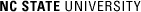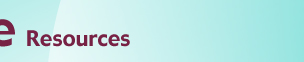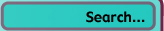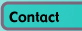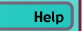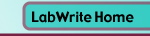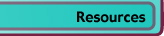# Graphing with Excel

## Basic Graphing in Excel

### Introduction

Beer's Law states that there is a linear relationship between concentration of a colored compound in solution and the light absorption of the solution. This fact can be used to calculate the concentration of unknown solutions, given their absorption readings. First, a series of solutions of known concentration are tested for their absorption level. Next, a scatter plot is made of this empirical data.

### Entering and Formatting the Data in Excel

Open Excel and begin by formatting the spreadsheet cells so the appropriate number of decimal places are displayed (see Figure 1a).

• Click and drag over the range of cells that will hold the concentration data (A5 through A10 for the sample data)
• Choose Format > Cells... (this is shorthand for choosing Cells... from the Format menu at the top of the Excel window)
• Click on the Number tab
• Under Category choose Number and set Decimal places to 5
• Click OK
• Repeat for the absorbance data column (B5 through B10 for the sample data), setting the decimal places to 4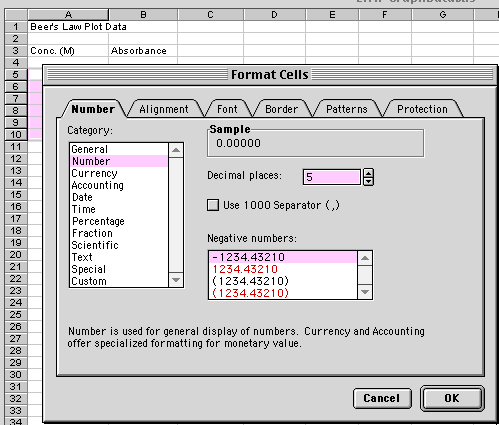Figure 1a.

Your data will go in the first two columns in the spreadsheet. Type what is seen in Figure 1b in the appropriate cells.

• Title the spreadsheet page in cell A1
• Label Column A as the Concentration (M) of the known solutions in cell A3. This is the independent variable
• Label Column B as the Absorbance readings for each of the solutions in cell B3. This is the dependent variable
• Enter the independent and dependent variable values
• Finally, enter the information shown in rows 12 and 13. These are absorbance values from two samples of unknown concentrations (more on this later).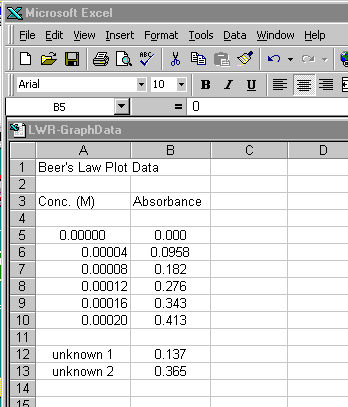Figure 1b.

The concentration data is probably better expressed in scientific notation.

• Highlight the concentration data and choose Format > Cells....
• Choose the Scientific Category and set the Decimal places to 2.

The last step before creating the graph is to choose the data you want to graph.

• Highlight the data in both the concentration and absorbance columns (but not the unknown data)

This is shown in Figure 2.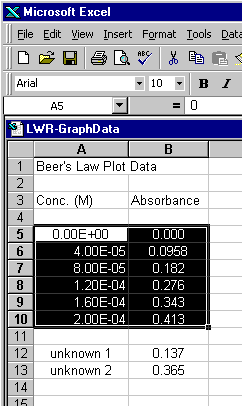Figure 2.

### Creating the Initial Scatter Plot

With the data you want graphed highlighted, start the chart wizard

• Choose the Chart Wizard icon from the tool bar (see Figure 3 for two examples). If the Chart Wizard is not visible, you can also choose Insert > Chart...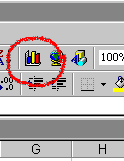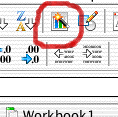Figure 3.

The first dialogue of the wizard comes up

• Choose XY (Scatter) and the unconnected points icon for the Chart sub-type (Figure 4a)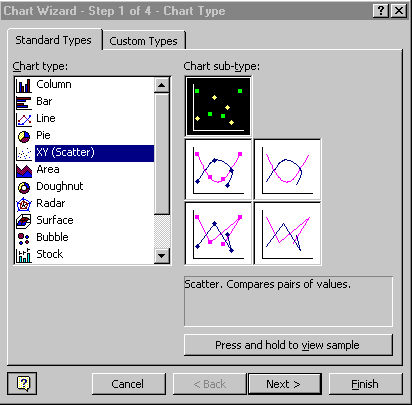Figure 4a.

• Click Next >

The Data Range box should reflect the data you highlighted in the spreadsheet. The Series option should be set to Columns, which is how your data is organized (see Figure 4b).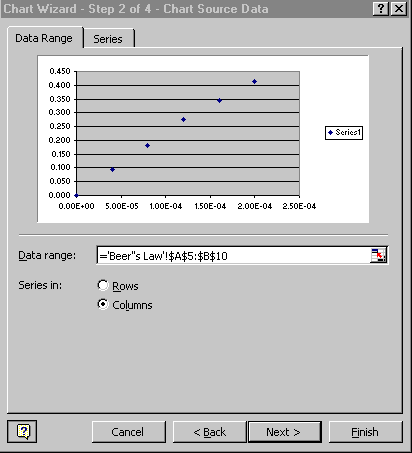Figure 4b.

• Click Next >

The next dialogue in the wizard is where you label your chart (Figure 4c)

• Enter Beer's Law for the Chart Title
• Enter Concentration (M) for the Value X Axis
• Enter Absorbance for the Value Y Axis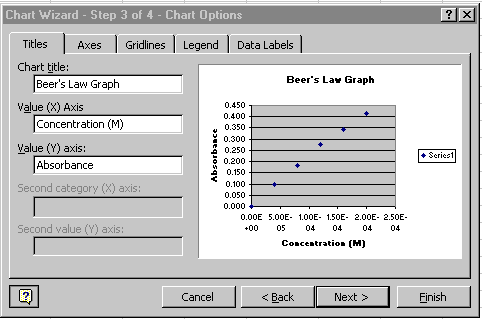Figure 4c.

• Click on the Legend tab
• Click off the Show Legend option (Figure 4d)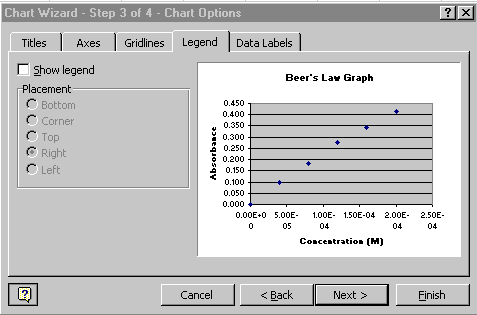Figure 4d.

• Click Next >

Keep the chart as object in Sheet 1 (the current sheet). See Figure 4e.Figure 4e.

• Click Finish

The initial scatter plot is now finished and should appear on the same spreadsheet page (called a sheet) as your original data. Your chart should look like Figure 5. A few items of note:

• Your data should look as though it falls along a linear path
• Horizontal reference lines were automatically placed in your chart, along with a gray background
• Your chart is highlighted with square 'handles' on the corners. When your chart is highlighted, a special Chart floating palette should also appear, as is seen in Figure 5. Note: If the Chart floating palette does not appear, go to Tools>Customize..., click on the Toolbars tab, and then click on the Chart checkbox. If it still doesn't show up as a floating palette, it may be 'docked' on one of your tool bars at the top of the Excel window.

With your graph highlighted, you can click and drag the chart to a wherever you would like it located on the spreadsheet page. Grabbing one of the four corner handles allows you to resize the graph. Note: the graph will automatically adjust a number of chart properties as you resize the graph, including the font size of the text in the graph. You may need to go back and alter these properties. At the end of the first part of this tutorial, you will learn how to do this.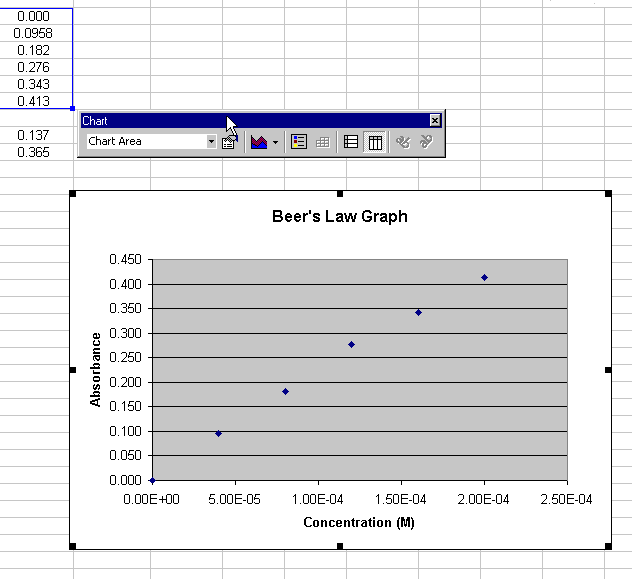Figure 5.

Go to the tutorial on creating regression lines to find out how to use a regression line with this scatter plot to calculate the concentrations of the two unknowns.

### Creating an Initial Scatter Plot of Titration Data

In this next part of the tutorial, we will work with another set of data. In this case, it is of a strong acid-strong base titration (see Figure 10 for the final plot). With this titration, a strong base (NaOH) of known concentration is added to a strong acid (also of known concentration, in this case). As the strong base is added to solution, its OH- ions bind with the free H+ions of the acid. An equivalence point is reached when there are no free OH- nor H+ ions in the solution. This equivalence point can be found with a color indicator in the solution or through a pH titration curve. This part of the tutorial will show you how to do the latter.

Note that there should be two columns of data in your spreadsheet:

Column A: mL of 0.1 M NaOH added

Column B: pH of the 0.1 M HCl / 0.1M NaOH mixture

• Using a new sheet in the spreadsheet workbook, enter your titration data as shown in Figure 6.
• Go to the Data Input Tutorial if you need hints on formatting the cells to the proper number of decimal places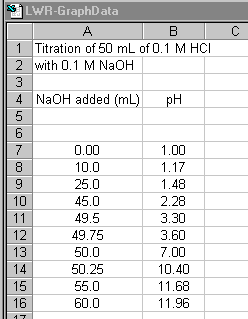Figure 6.

Now, create a scatter plot of titration data, just as you did with the Beer's Law plot (Figure 7).

• Highlight the titration data and the Column headers
• Click on the Chart wizard icon
• Choose XY (Scatter) and the Scatter Chart sub-type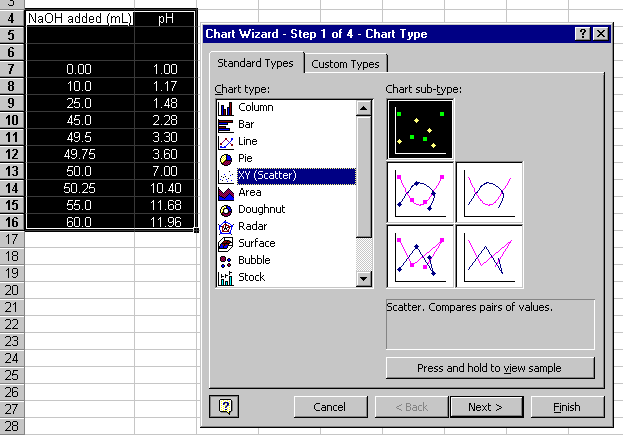Figure 7.

Continue through steps 2 through 4 of the Chart wizard:

• The defaults for step 2 should be fine if you properly highlighted the data
• In step 3 enter the chart Title and x and y axis Labels and turn Off the Legend
• In step 4, leave as an object in the current page

The resulting plot should look like Figure 8: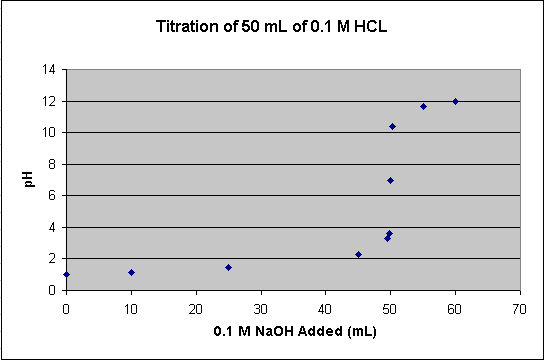Figure 8.

### Changing the Scatter Plot to a Line Graph

All of the points of the titration data can be connected to form a smooth curve. With this approach, the curve is guaranteed to go through all of the data points. This option can be used if you have only one pH reading per amount of NaOH added. If you have multiple pH readings for each amount added on the scatter plot, you will not end up with a smooth curve. To change the scatter plot to a (smoothed) line graph (Figure 9):

• Choose Chart > Chart Type...
• Select the Scatter connected by smooth lines Chart subtype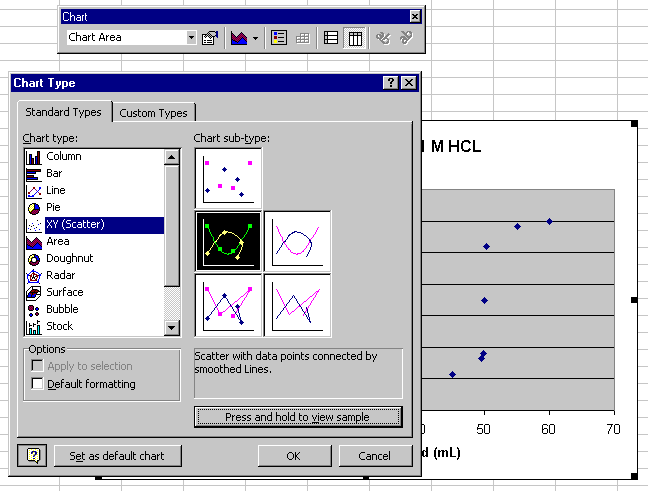Figure 9.

The result should look like Figure 10: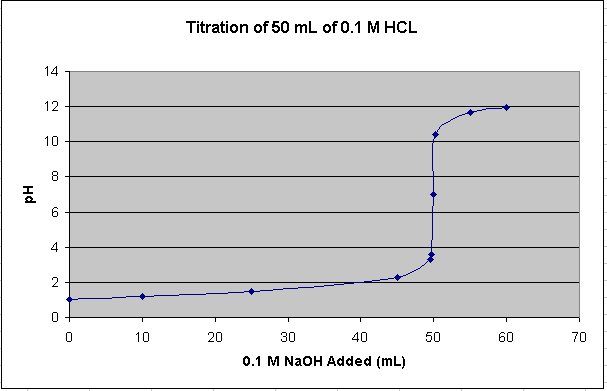Figure 10.

This smooth, connected curve helps locate where the steep part of the curve passes through pH 7.© Copyright NC State University 2004 Sponsored and funded by National Science Foundation (DUE-9950405 and DUE-0231086) Site design by Rosa Wallace Rev. RW 5/16/05